Next: Partial results from , Up: Inferring and of the Previous: Introduction

# Uncertain constraints

The relations (1)-(4) of Ref.  can be written in the following way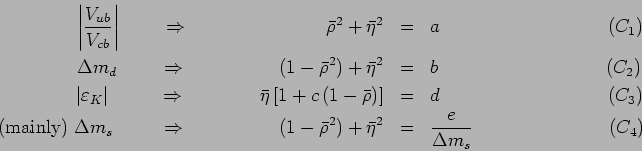where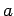,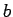, ...are the constraint parameters, functions of many quantities; the most crucial of those determined experimentally are indicated on the left hand side of the equations. Note that the order of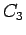and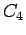is exchanged with respect to Eqs. (3)-(4) of Ref. . This is because, since the present information concerning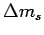is of different quality with respect to the other quantities, the constraintneeds a more careful treatment than the others, and it will be introduced after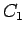-. This is also the reason whyappears explicitly in the constraint.

In the ideal case all parameters are perfectly known, and the constraints would give curves in the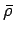-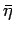plane. For example,would give a circle of radius. In other terms, due to this constraint all points of the circumference would be appear to us likely, unless there is any other experimental piece of information (or theoretical prejudice) to assign a different weight to different points. In the ideal case the p.d.f. describing our beliefs in theandvalues would be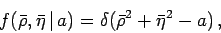(1)

with the Dirac delta meaning the limit of a very narrow p.d.f. concentrated on the circumference. Similar arguments hold for the parameters of the other constraints. Let us call them generically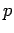(the fact thatdepends on two parameters is conceptually irrelevant).

In the real caseitself is not perfectly known. There are values which are more likely, and values which are less likely, classified by the p.d.f.. This means that, e.g. for the parameter, we deal with an infinite number of circles, each having its weight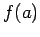. It follows that the points of the (,) plane get different weights.

The probability theory teaches us how to evaluate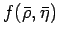taking into account all possible values of: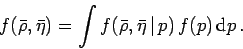(2)

Now the problem becomes how to evaluate, knowing that eachdepends on many input quantities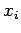, denoted all together with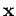and described, in the most general case, by a joint p.d.f.. In most cases of practical interest, including the one we are discussing, eachcan be considered independent from the others, and the joint distribution simplifies to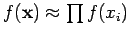. Calling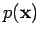the function which relatesto,can be generally obtained as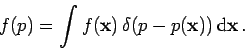(3)

This integral, as well as the previous integrals, is usually performed by Monte Carlo techniques. The calculations can be simplified in the following way.
• First, we rely on the central limit theorem, assumingto be Gaussian for all parameters (but with no constraint on the shape of!).
• Expected value, standard (deviation) uncertainty and correlation are basically obtained by the usual propagation (see Ref.  for a similar application).
• Non linear effects in the propagation have been taken into account up to second order, using formulae of Ref.  (see this paper for the practical modeling of uncertainties and treatment of asymmetric cases).
• The correlation between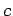and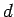has been taken into account building a bi-variate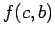.

As input quantities, Table 1 of Ref.  is used, combining properly (i.e. quadratically) the standard deviations. For example, for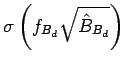expressed in MeV we obtain, where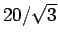stands for the standard deviation of a uniform distribution of half width 20 MeV. Note that, contrary to what some authors critical about Ref.  (and the related papers and presentations to conferences) think, I have the impression that my colleagues tend to make slightly conservative assessments of uncertainties. This feeling that I had a priori discussing with them is somewhat confirmed a posteriori by the excellent overlap of the partial inference by each constraint (as it will be shown in Fig. 9) and self-consistency between input parameters and values coming out of the inference obtained without the their contribution (see Ref. ).

The following results are obtained in terms of expected values and standard deviations (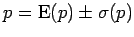''):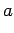(4)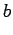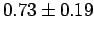(5)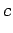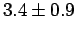(6)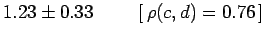(7)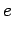(8)

Only the correlation betweenandis relevant, and all others can be neglected, being below the 10% level (even that betweenand, related by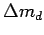, is negligible, being only +7%). The radii of the circles given byand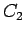areand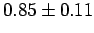, respectively. These radii have the meaning of the sides of the unitarity triangle opposite to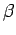and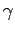, respectively, provided byandalone.Next: Partial results from , Up: Inferring and of the Previous: Introduction
Giulio D'Agostini 2004-01-20